## Resolution Equation in HPLC

NOTE: Figure and equation numbers are shown by moving over the figure or the equation. They are not shown separately.

On this page, I want to deal with the different formulations for a resolution used in HPLC and explain the subtle differences between the formulas that are used. These explanations have been taken from an article by A.S. Said (J. High-Resolution Chromatography &Chromatography Communications, (1979) 193).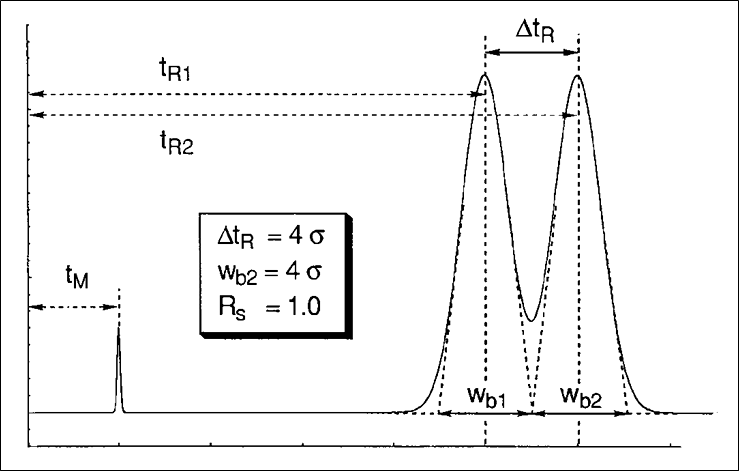Figure 1 shows the essential parameters to measure resolution in HPLC. The formula for resolution is given below.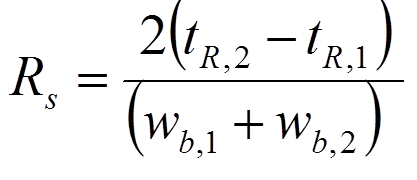resulting in resolution = 1 after substituting the values given in the figure on the left.

The issue described here is that in simplification of equation 1.1, different assumptions can be made which lead to different expressions for resolution in chromatographic parameters.

In a first approach, issued by Purnell (J. Chem. Soc., (1960) 1268), it is assumed that the width of the first peak equals the width of the second peak. After some algebraic manipulations, one arrives at equation 1.2:In equation 1.2. N2 is the plate number of the second peak, k2 is the retention factor of the second peak and alpha is the selectivity factor defined as the ratio of k2 and k1. However, when one assumes that the peak width of the second peak equals the width of the first peak one arrives at equation 1.3 which is known as the Knox equation for resolution: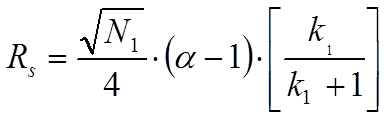It is imperative to realize the difference between these two equations for the resolution of chromatographic peaks. Many mistakes are easily observed especially in non-peer reviewed journals and in promotional literature from HPLC column manufacturers. In most cases, the indices are omitted. This implies that the average plate number and average of k1 and k2 are used. In fact, however, in case the average values of N and k of the two peaks under review according to Said (JHRCCC, (1979) 193) the resolution equation changes to:More details can be found in the publication by Prof. Pat Sandra, J. High-Resolution Chromatography, 12, 82 (1989).

In a very detailed and informative review, Prof. Joe Foley has expanded this topic further and has provided additional accurate formulations of the resolution equation (Table 1, eqn. 9-11 in J.P. Foley, Analyst 116, 1275 (1991). Foley has emphasized the assumptions that lay at the base of the different resolution equations. He eventually proposed three additional equations for the resolution of chromatographic bands eluting from the column.

## Definition of the permeability of a packed bed

The definition of permeability of a packed (U)HPLC column is frequently inappropriately and incorrectly used in many popular and column manufacturer publications. This short article and the pages in the submenus are intended to clarify this area.

In prinicple, the permeability of a packed (U)HPLC column is defined as the proportionality factor in the relation between velocity of the solvent in the column and pressure drop.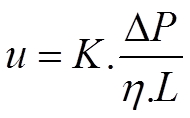Here u is the average solvent velocity, ΔP the net pressure drop over the column, η the viscosity of the solvent and L the length of the column. The proportionality factor K has the dimension m^2 .

Two questions however pop up:

1. How is the solvent velocity defined?
2. How does K relate to the porous structure of the packed particle bed?

This will be addressed in the following pages of this blog.

## Permeability Equations - Superficial Velocity

The definitions provided here are derived from two manuscripts:

G. Deininger, Strömungvorgänge in Säulen und ihre Bedeutung in der Säulenchromatographie,
Ber. Bunsengesellschaft für physikalische Chemie, 77, 145 (1973).

C.A. Cramers, J.A. Rijks, J.P.M. Schutjes, Factors determining flow rate in chromatographic columns,
Chromatographia, 14, 439-444, (1981).

In chemical engineering, the solvent velocity is defined as (lhs in equation 2.2). This is called superficial velocity.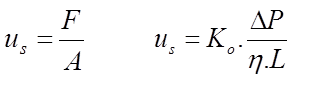F is the volumetric flow rate through the column and A the cross-sectional surface of the empty column. In equation 2.2, Ko is called "specific permeability". This formulation is also known as Darcy's law.

However other definitions of solvent velocity are used in this context and need to be explained.

## Permeability Equations - Interstitial Velocity

It is defined as the velocity obtained by flow through the column between the particles. The free volume in the column available for flow is given by the left-hand equation below (equation 2.3):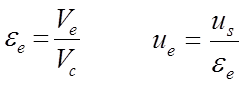where Ve is the volume between the particles and Vc is the volume of the empty column. The interstitial solvent velocity ue relates to the superficial solvent velocity as given in the rhs of equation 2.3.

These definitions of solvent velocity come from chemical engineering. In chromatography, a third definition of solvent velocity is used mostly viz. the chromatographic solvent velocity. This velocity is easily determined as the quotient of the column length Lc and the hold-up time of an unretained solute t0 (equation 2.4).

## The Kozeny Carman Equation

The Kozeny–Carman equation is used in the field of fluid dynamics to calculate the pressure drop of a fluid flowing through a packed bed of solid particles. This equation relates the permeability of tube packed with particles to interparticle porosity, bed morphology and particle size and shape. The model replaces the space between the particles as a set of capillaries and accounts for the difference in length of the hypothetical capillaries (equation 2.8).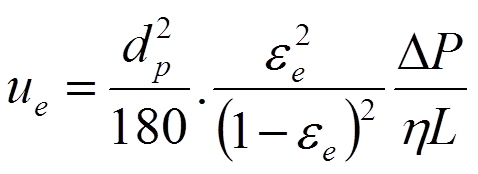## Chromatographic Column Resistance Factor

The equations on the previous page originate from chemical engineering and geology disciplines. As mentioned on a previous page, in the early days, chromatographers took a slightly different approach by using chromatographic solvent velocity since it can be measured easily. Chromatographic permeability (J.H. Knox et al., Chromatographia, 10, 279 (1976)) was defined as given in equation 2.7 on a previous page and shown here again: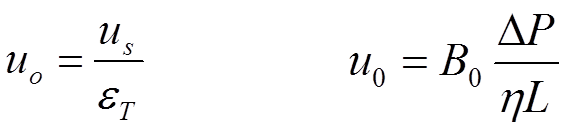Substitution of the lhs part of the K-C equation (2.9 or 2.10)  leads to equation 2.11: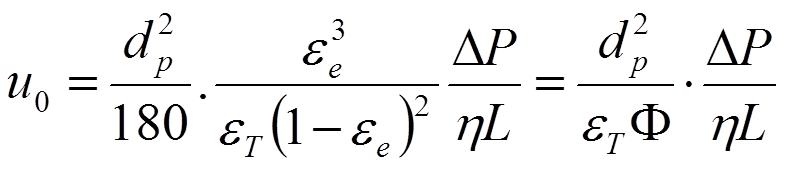Knox defined the chromatographic column resistance factor Φ0 (equations 2.12) as: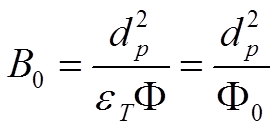A superior quality HPLC column will have a chromatographic column resistance factor Φ0 around 700 which means an interparticle porosity of 0.4 and a total porosity of 0.7.

Again, it is imperative to note the differences between the solvent velocity definitions used and the column permeability derived from it. The indices are important.